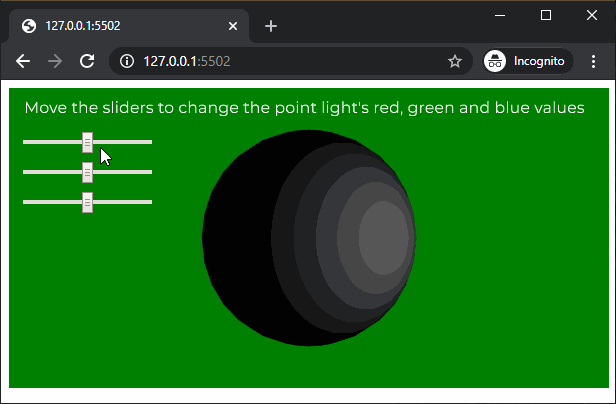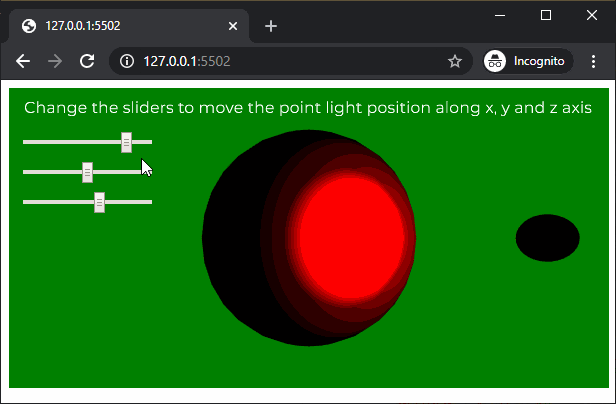# p5.js | pointLight() Function

• Last Updated : 27 Apr, 2020

The pointLight() function in p5.js is used to create a point lights with the specified color and position in the scene. A scene can have a maximum of 5 active point lights at a time.

Syntax:

Hey geek! The constant emerging technologies in the world of web development always keeps the excitement for this subject through the roof. But before you tackle the big projects, we suggest you start by learning the basics. Kickstart your web development journey by learning JS concepts with our JavaScript Course. Now at it's lowest price ever!

`pointLight( v1, v2, v3, x, y, z )`

OR

`pointLight( v1, v2, v3, position )`

OR

`pointLight( color, x, y, z )`

OR

`pointLight( color, position )`

Parameters: This function accept eight parameters as mentioned above and described below:

• v1: It is a number which determines the red or hue value relative to the current color range.
• v2: It is a number which determines the green or saturation value relative to the current color range.
• v3: It is a number which determines the blue or brightness value relative to the current color range.
• x: It is a number which determines the x axis position of the light.
• y: It is a number which determines the y axis position of the light.
• z: It is a number which determines the z axis position of the light.
• position: It is p5.Vector which defines the position of the light.
• color: It is a color string or p5.Color which defines the color of the point light.

Below example illustrates the pointLight() function in p5.js:

Example 1:

 `let newFont;``let directionalLightEnable = ``false``;`` ` `function` `preload() {``  ``newFont = loadFont(``'fonts/Montserrat.otf'``);``}`` ` `function` `setup() {``  ``createCanvas(600, 300, WEBGL);``  ``textFont(newFont, 16);`` ` `  ``redSlider = createSlider(0, 255, 128, 1);``  ``redSlider.position(20, 50);`` ` `  ``greenSlider = createSlider(0, 255, 128, 1);``  ``greenSlider.position(20, 80);`` ` `  ``blueSlider = createSlider(0, 255, 128, 1);``  ``blueSlider.position(20, 110);``}`` ` `function` `draw() {``  ``background(``'green'``);``  ``text(``"Move the sliders to change the point light's red, "``+``                        ``"green and blue values"``, -285, -125);``  ``noStroke();`` ` `  ``redValue = redSlider.value();``  ``greenValue = greenSlider.value();``  ``blueValue = blueSlider.value();`` ` `  ``// Create a point light with the selected light color``  ``pointLight(redValue, greenValue, blueValue, 100, 0, 150);`` ` `  ``ambientMaterial(255);`` ` `  ``// Create the sphere on which the point light will work``  ``sphere(100);``}`

Output:Example 2:

 `let newFont;``let directionalLightEnable = ``false``;`` ` `function` `preload() {``  ``newFont = loadFont(``'fonts/Montserrat.otf'``);``}`` ` `function` `setup() {``  ``createCanvas(600, 300, WEBGL);``  ``textFont(newFont, 16);`` ` `  ``xPosSlider = createSlider(-150, 150, 100, 1);``  ``xPosSlider.position(20, 50);`` ` `  ``yPosSlider = createSlider(-300, 300, 0, 1);``  ``yPosSlider.position(20, 80);`` ` `  ``zPosSlider = createSlider(0, 250, 150, 1);``  ``zPosSlider.position(20, 110);`` ` `}`` ` `function` `draw() {``  ``background(``'green'``);``  ``text(``"Change the sliders to move the point light position "``+``                          ``"along x, y and z axis"``, -285, -125);``  ``noStroke();`` ` `  ``xPositionValue = xPosSlider.value();``  ``yPositionValue = yPosSlider.value();``  ``zPositionValue = zPosSlider.value();`` ` `  ``// Create a point light at the selected location``  ``pointLight(255, 0, 0, xPositionValue, yPositionValue, zPositionValue);`` ` `  ``// Create a sphere to show the demonstrate``  ``// of the point light location``  ``translate(xPositionValue, yPositionValue, zPositionValue);``  ``sphere(10);``  ``translate(-xPositionValue, -yPositionValue, -zPositionValue);`` ` `  ``specularMaterial(255);`` ` `  ``// Create the sphere on which the point light will work``  ``sphere(100);``}`

Output:Online editor: https://editor.p5js.org/

My Personal Notes arrow_drop_up